# Quiz 12: Decision Analysis

Business

Decision tree This is problem solving or decision-making strategy where a business problem s formulated in a tree like structure and solved under different conditions with probabilities. Construct a decision tree for evaluating R D of WSP Company.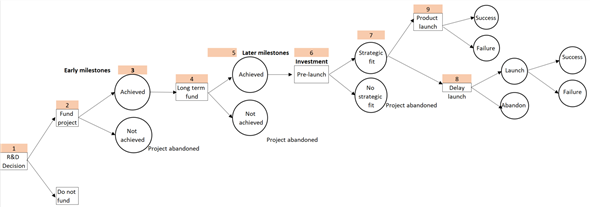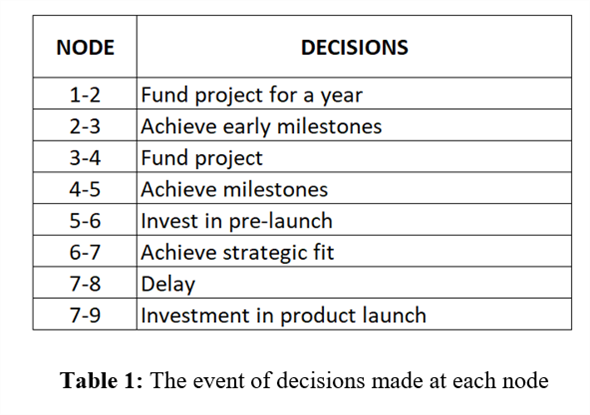Consider the possibilities of the costing of each project to obtain an estimated value. Expected Value is the probability weighted value of all the possible outcomes.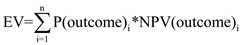Calculate the expected value and rank the project by using the formula in eq. (1)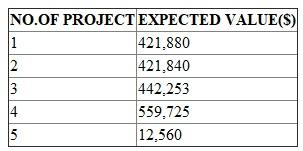Maximax Criterion - Select the maximum payoff for each decision. Then, select the maximum of those maximum payoffs. Maximin Criterion - Select the minimum payoff for each decision. Then, select the maximum of those minimum payoffs. Consider the following information as shown below: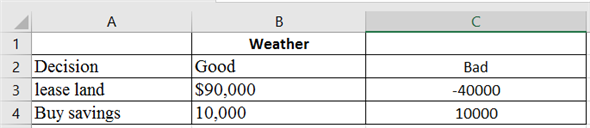a. Calculate the maximum cost decision by using the following formulas in the excel as shown below: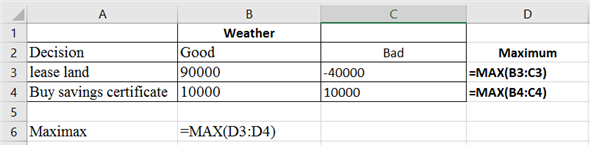Following values will be obtained: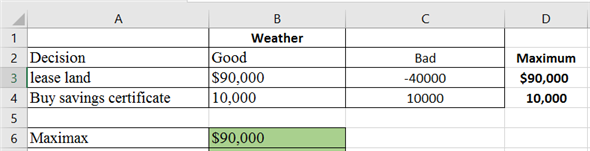Therefore, the best decision using the maxi-max approach is lease land. b. Calculate the maximum of minimum cost decision by using the following formulas in the excel as shown below: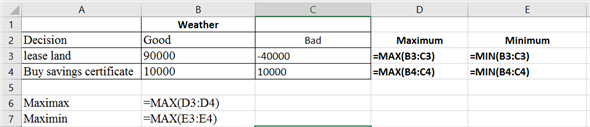Following values will be obtained: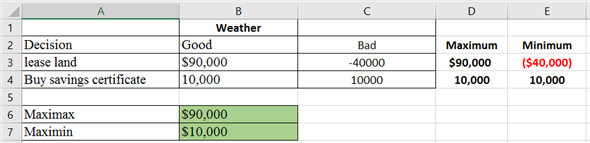Therefore, the best decision using the maximin approach is buy saving certificate.

Overview: SA Inc. is a property development firm who purchased an old house near the town square in CC Falls area near to the State University. The problem aroused when the SA Inc., wanted to build a new high-density student apartment demolishing this house after the lease with state university expired. This was opposed by the town council. The SA Inc. had developed three options to gain permit in building apartment in the proposed area. SA Inc. has estimated all the probabilities of problems that could occur if they chose any of the three options. An optimal appropriate decision is to be recommended. A. The decision tree for the probability building a high-density apartment is as follows: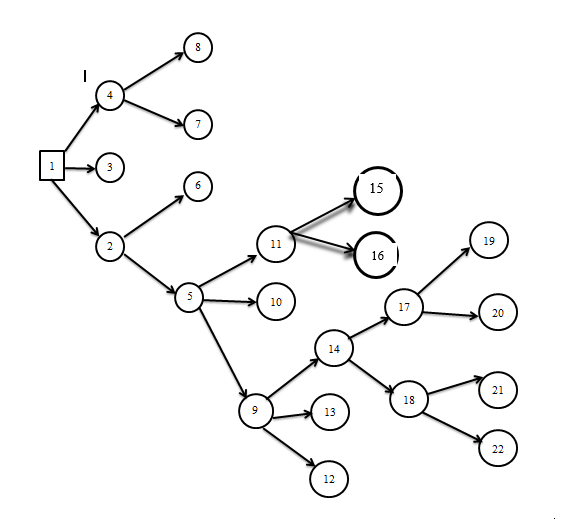The decisions at the respective nodes are given below: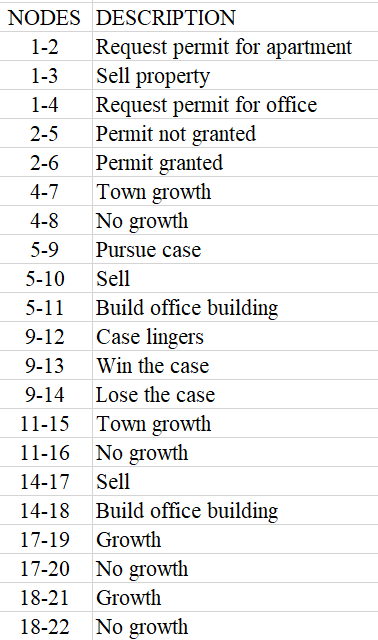The decision tree analysis can be explained with the below tables: Table 1: Analysis before the event occurred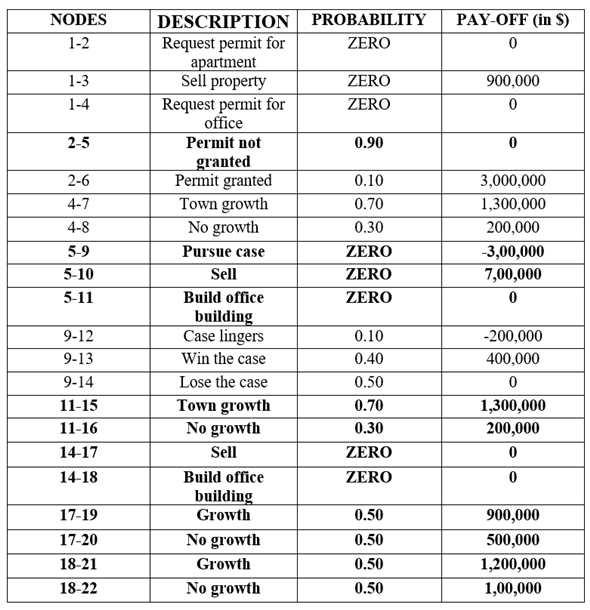Calculate the payoff at each node by calculating the expected value thereby considering the respective probability. Calculate Pay-off for Node 1-4 as follows:Similarly, calculate expected values for Nodes 1-3 and 1-2 and proceed.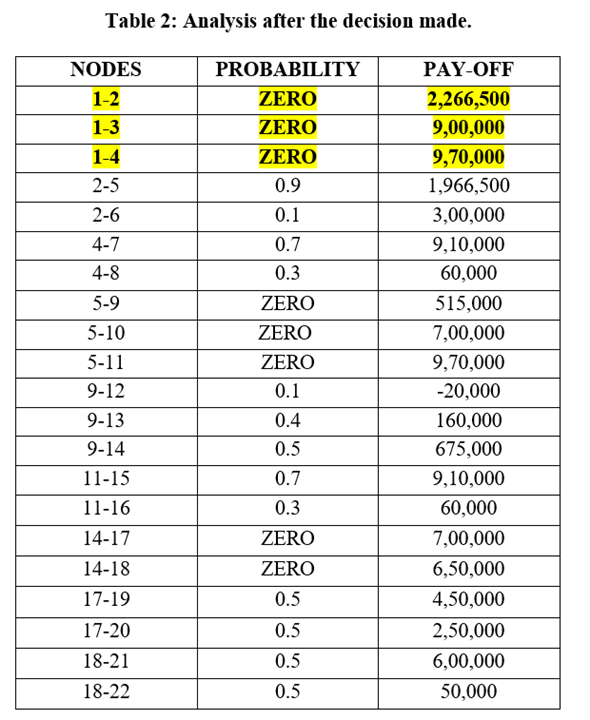B. The pay-off of 'Request a permit for the apartment' is valued to \$2,266,500. Since this pay-off expected value is highest, thus decision of going with request permit for the constructing the building should be considered.

There is no answer for this question

There is no answer for this question

There is no answer for this question